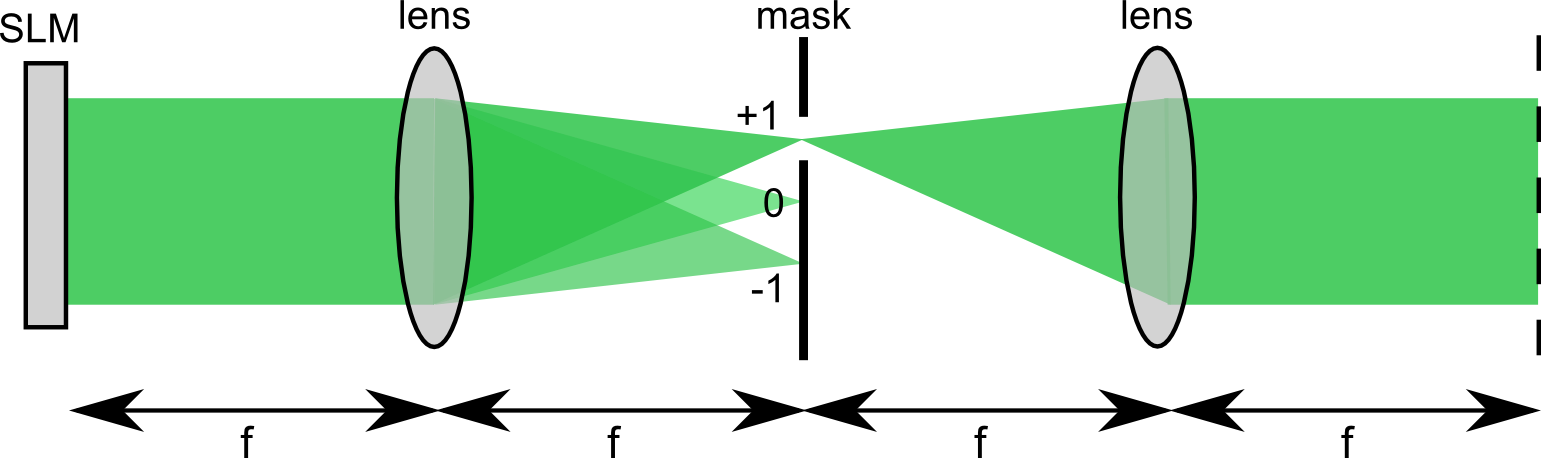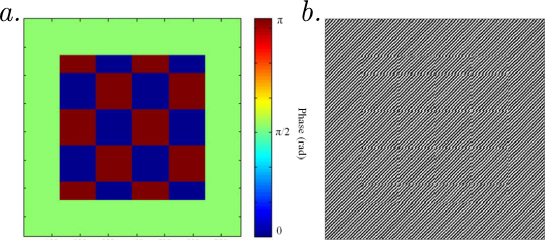##The optical field measured at the output of a complex medium (multimode waveguide, multimode cavity, scattering medium...) is the result of the interference of the many paths taken by the light. Most of the applications of wavefront shaping techniques in these media rely on taking advantage of these interference effects to force the medium to perform a desired function. For this reason, the phases of the controlled segments of the input wavefront are usually the most important degrees of freedom to control. Common phase-only Spatial Light Modulators (SLMs) have a limited refresh rate (~100 Hz) due to the liquid crystal technology. This limits the applications in media with a low decorrelation time (like biological tissues) or for experiments for which a long optimization process is needed. Although a new class of phase-only SLMs based on deformable mirrors allows high-speed modulations (>30 kHz), these devices are currently very expensive compared to liquid crystal technology and have a limited resolution (~1000 pixels maximum). On the other hand, fast binary-amplitude modulators with high resolutions are widespread and affordable since the technology is the same one as used in commercial digital projectors. In a recent publication, [D.B. Conkey et al., Opt. Express (2012)] introduced a method to use such a binary-amplitude Deformable Mirror Device (DMD) for phase modulation. This technique allows a fast phase modulation (up to 23 kHz) at the cost of a loss of resolution.

Some manufacturers of Digital Light Processing (DLP) projectors offer the possibility to purchase development kits with an SDK that allows us to directly control the DMD chipset with a computer. The only one widely available is the Texas Instrument DLP Discovery 4100 Development Kit compatible with two different chipsets; - the DLP7000 with a resolution of 1024x768, a 0.7-inch chipset and a maximum refresh rate of 32.5 kHz - the DLP9500 with a resolution of 1920x1080, a 0.95-inch chipset and a maximum refresh rate of 23.1 kHz.

This technology is based on micro-mirrors that allows a binary amplitude modulation, meaning that the amplitude of one pixel can only be set to 0 or 1, with no control on the phase. To get phase modulation using an amplitude modulator, the idea is to filter an amplitude signal in the Fourier plane to obtain a complex field modulation. Let $$\phi(x,y)$$ be the phase hologram to display. The Lee method [W.H. Lee, Progress in Optics (1978)] consists of generating the amplitude pattern:

$$f(x,y) = \frac{1}{2}\left[ 1+cos(2\pi (x-y) \nu_0 -\phi(x,y))\right]$$

This function can also be written:

$$f(x,y) = \frac{1}{2} + \frac{1}{4}e^{i 2\pi (x-y) \nu_0} e^{-i \phi(x,y)} + \frac{1}{4}e^{i 2\pi (y-x) \nu_0} e^{i \phi(x,y)}$$

The three terms of this expression have respective center spatial frequencies $$(\nu_x=0,\nu_y=0)$$, $$(\nu_x=\nu_0,\nu_y=-\nu_0)$$ and $$(\nu_x=-\nu_0,\nu_y=\nu_0)$$. If $$\nu_0$$ is higher than the highest spatial frequency of $$\phi(x,y)$$, these three terms correspond to three distinct orders of diffraction, that we call 0, +1, and -1. We see that the last component of the previous expression corresponds to the desired phase modulation tilted by an angle corresponding to the diffraction. To select only this term, one can use a 4-f system with an iris or a pinhole in the Fourier plane [see Fig. 1.].Figure 1. Schematic of the setup.

The resulting field after the 4-f system is proportional to $$e^{i\phi(x,y)}$$.

Since DLP devices can only perform a binary amplitude modulation, we generate a binary amplitude hologram by thresholding the previous amplitude hologram:

$$g(x,y)= \,\,\,\begin{array}{l} 1 \,\,\text{if} \,\,f(x,y)>1/2 \\ 0 \,\,\text{otherwise} \end{array}$$

We show in Fig. 2 an example of binary hologram used to generate a phase pattern.Figure 2. Example of a desired phase pattern (a.) and the corresponding binary Lee hologram which encodes the same phase distribution. Image from [D.B. Conkey et al., Opt. Express (2012)].

The phase of the desired field is encoded in the phase shift of the cosine function in $$f(x,y)$$. For a given spatial sampling, the approximation $$g(x,y)$$ for the binary amplitude modulation is less efficient to render the desired phase pattern since the phase shift is partially blurred by the square shape of the signal. To obtain a correct approximation of the phase profile, it is necessary to have a sufficient number of oscillations inside one macro-pixel. This requires a great number of pixels per macro-pixel, which drastically decreases the resolution of the system.

The three parameters to carefully tune to obtain a good trade-off between resolution and fidelity of the generated phase pattern are the carrier spatial frequency $$\nu_0$$, the spatial sampling (how many pixels per macro-pixel) and the diameter of the iris or pinhole (that defines the cutoff of the spatial frequency filtering).

Created by sebastien.popoff on 20/06/2013